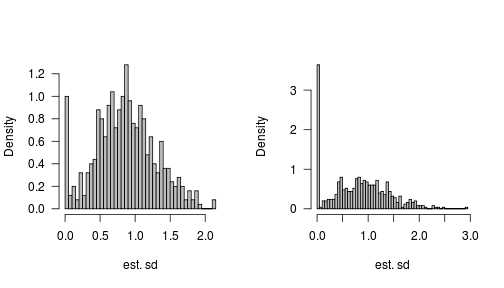# Mixed model simulations

What happens if we try fit mixed models with too few samples? Here's a little example.

Let's consider just two-level mixed (actually pure random-effect) models, for simplicity.

A function to simulate data and one to fit the model and extract the estimated random-effect standard deviation. (This is done with development lme4 but the results would probably be nearly identical in stable lme4 or in nlme)

simfun <- function(n1 = 5, n2 = 5, sd1 = 1, sd2 = 1) {
d <- expand.grid(f1 = factor(seq(n1)), f2 = factor(seq(n2)))
u1 <- rnorm(n1, sd = sd1)
d\$y <- rnorm(n1 * n2, mean = u1, sd = sd2)
d
}
require(lme4)
fitfun <- function(d = simfun()) {
sqrt(unlist(VarCorr(lmer(y ~ (1 | f1), data = d))))
}


Run 500 sims with n1=5 samples at the top level and 500 with n1=3

set.seed(101)

sd_dist1 <- replicate(500, fitfun())
sd_dist2 <- replicate(500, fitfun(simfun(n1 = 3)))
sd_List <- list(n1.5 = sd_dist1, n1.3 = sd_dist2)


Histograms with slightly-prettier-than-default settings:

plotfun <- function(x, trueval) {
par(las = 1, bty = "l")
hist(x, breaks = 50, col = "gray", main = "", xlab = "est. sd", freq = FALSE)
}


Plot results:

par(mfrow = c(1, 2))
invisible(lapply(sd_List, plotfun))We can see that there is a spike at zero in both cases, although it is much larger when n1=3. Proportion of samples exactly equal to zero:

sapply(sd_List, function(x) mean(x == 0))

##  n1.5  n1.3
## 0.048 0.182


If we were lucky (in some sense), these zeros would be counterbalanced by large values so that we could say the overall estimate was unbiased (i.e., the mean estimate was equal to the true value, even if the estimates varied all over the place).

This isn't true though. Here we compute the mean, standard error, and confidence intervals ($$\pm 2 \text{SEM}$$) for both runs:

sfun <- function(x) {
r <- list(mean = mean(x), sem = sd(x)/sqrt(length(x)))
r <- with(r, c(r, list(lwr = mean - 2 * sem, upr = mean + 2 * sem)))
unlist(r)
}
print(s_tab <- sapply(sd_List, sfun), digits = 3)

##        n1.5   n1.3
## mean 0.8615 0.8051
## sem  0.0192 0.0259
## lwr  0.8231 0.7534
## upr  0.8999 0.8569

bias_pct <- round((1 - s_tab["mean", ]) * 100)


n1=5 is biased low by 14%; n1=3 is biased low by 19%.

And by the way, this is with the default REML=TRUE setting. I would also guess that this is generic behaviour of modern mixed models, not a problem with R's code – I would guess you'd get the same sorts of results from ASREML/Genstat/SAS PROC MIXED etc.